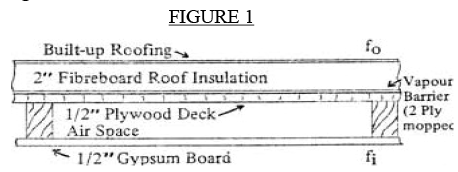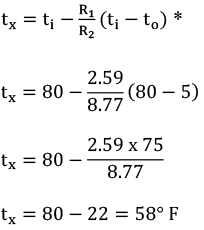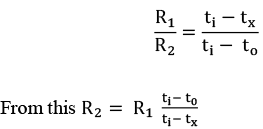# R? U? Sufficient Insulation to Control Dew Point Temperature at Vapour Barrier

August 1969

In our May, 1969, “Good Roofing” technical bulletin titled “Common Technical Terms and Quantities used in Calculating Insulation Values,” we listed certain terms and values in common use. Using that data, the intent of this bulletin is to put that information to practical use, in simplified form.

Problem: 1

Determine the overall resistance and the overall coefficient U through a roof section, as shown in Figure 1.Solution: 1R1 = the resistance from the inside air to any point in the structure at which the temperature is to be determined.

R2 = the overall resistance from inside air to outside air.

ti = inside air temperature

to = outside air temperature.

tx = temperature to be determined.

Problem: 2

Reference the same roof section (Figure 1) determine the temperature at the vapour barrier if the inside temperature were 80°F, and the outside temperature were + 5°F.

Solution: 2*NOTE If the to were below 0°F the minus — sign between ti and to would be changed to a plus + sign.

Now consider other humidity/condensation problems.

The nomograph (Figure 2) may be used to determine the dew point when the (dry bulb) air temperature and the percentage of relative humidity are known.

Problem: 3

If the inside air temperature is 90°F and the relative humidity is 40%, what is the dew point temperature?

Solution: 3

Place a ruler across the three vertical lines of the nomograph, so that it crosses the A line at 90 and C at 40, then read the dew point temperature On B, which is approximately 63°F.

Problem: 4

If the inside temperature is 85°F and the temperature at the vapour barrier is 60°F, what maximum percentage of relative humidity may be held in the building without moisture condensing within the roof deck?

Solution: 4

Place a ruler across the nomograph lines ABC so that it crosses A at 85 and B at 60. Read the answer on C, which is approximately 42% relative humidity.

Problem: 5

Assume a roof construction like the one shown in Problem l, determine the thickness of insulation for the following conditions so there will be no condensation at the vapour barrier

ti = 80°F
to = 5°F
RH = 40%

All other values are the same as in Problem 1.

Solution: 5

Using ti = 80°F and RH = 40% obtain from nomgraph

tx = 53°F

where tx is the dew point temperature in the room and can be assumed to be the same at the vapour barrier. Let Rx be resistance of insulation to be found.

Now the basic equation isAlso we know that R2 = Rx + 3.21 (since total value of resistances in Problem 1 excluding insulation is 3.21)Substituting the values known we get:From May 1969 Technical Bulletin R for l” of fibreboard is 2.78. Therefore thickness of fibreboard insulation isAlways choose the standard thickness of insulation next above the calculated thickness to ensure the dewpoint temperature occurs above the vapour barrier

Therefore T = 1 – ½”

In this example one might use 2″ of fibreboard insulation because 1 -1 /2” may be considered too close above the calculated thickness for safety.

NOTE: If the underside of the deck is exposed R1 is the sum of only the inside film coefficient (fi) and the resistance of the deck.